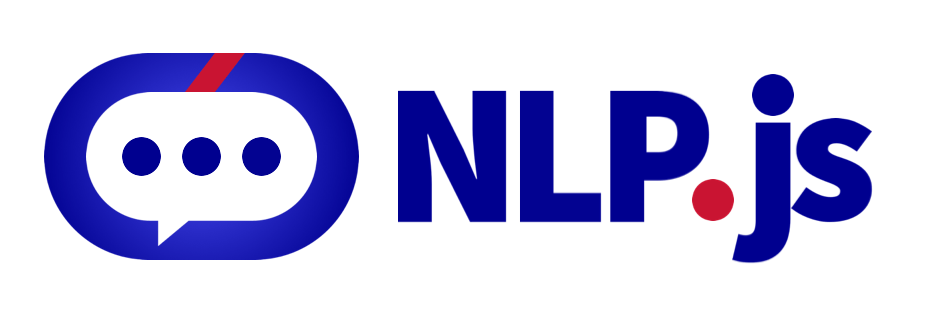# @nlpjs/neural

Neural Network

## Usage no npm install needed!

``````<script type="module">
import nlpjsNeural from 'https://cdn.skypack.dev/@nlpjs/neural';
</script>``````# NeuralNetwork

## Introduction

NeuralNetwork is the class for an NLU Neural Network, able to train a classifier and then classify into intents. This package is part of the NLP.js suite.

## Installing

NeuralNetwork is a class of the package @nlpjs/neural, that you can install via NPM:

``````  npm install @nlpjs/neural
``````

## Corpus Format

For training the classifier you need a corpus. The corpus format is an array of objects where each object contains an input and output, where the input is an object with the features and the output is an object with the intents:

``````[
{
"input": { "who": 1, "are": 1, "you": 1 },
"output": { "who": 1 }
},
{
"input": { "say": 1, "about": 1, "you": 1 },
"output": { "who": 1 }
},
{
"input": { "why": 1, "are": 1, "you": 1, "here": 1 },
"output": { "who": 1 }
},
{
"input": { "who": 1, "developed": 1, "you": 1 },
"output": { "developer": 1 }
},
{
"input": { "who": 1, "is": 1, "your": 1, "developer": 1 },
"output": { "developer": 1 }
},
{
"input": { "who": 1, "do": 1, "you": 1, "work": 1, "for": 1 },
"output": { "developer": 1 }
},
{
"input": { "when": 1, "is": 1, "your": 1, "birthday": 1 },
"output": { "birthday": 1 }
},
{
"input": { "when": 1, "were": 1, "you": 1, "borned": 1 },
"output": { "birthday": 1 }
},
{
"input": { "date": 1, "of": 1, "your": 1, "birthday": 1 },
"output": { "birthday": 1 }
}
]
``````

## Example of use

The file corpus.json should contain the corpus shown in the Corpus Format section for this example. This will train this corpus and run the input equivalent to the sentence "when birthday". The result is each intent with the score for this intent.

``````const { NeuralNetwork } = require('@nlpjs/neural');
const corpus = require('./corpus.json');

const net = new NeuralNetwork();
net.train(corpus);
console.log(net.run({ when: 1, birthday: 1 }));
// { who: 0, developer: 0, birthday: 0.7975805386427789 }
``````

## Exporting trained model to JSON and importing

You can export the model to a json with the toJSON method, and import a model from a json with fromJSON method:

``````const { NeuralNetwork } = require('@nlpjs/neural');
const corpus = require('./corpus.json');

let net = new NeuralNetwork();
net.train(corpus);
const exported = net.toJSON();
net = new NeuralNetwork();
net.fromJSON(exported);
console.log(net.run({ when: 1, birthday: 1 }));
``````

## Options

There are several options that you can customize:

• iterations: maximum number of iterations (epochs) that the neural network can run. By default this is 20000.
• errorThresh: minimum error threshold, if the loss is lower than this number, then the training ends. By default this is 0.00005.
• deltaErrorThresh: minimum delta error threshold, this is, the difference between the current and the last errors. If the delta error threshold is lower than this number, then the training ends. By default this is 0.000001.
• learningRate: learning rate for the neural network. By default this is 0.6.
• momentum: momentum for the gradient descent optimization. By default this is 0.5.
• alpha: Multiplicator or alpha factor for the ReLu activation function. By default this is 0.07.
• log: If is false then no log happens, if is true then there is log in console. Also a function can be provided, and will receive two parameters: the status and the elapsed time of the last epoch. By default this is false.

Example of how to provide parameters:

``````const { NeuralNetwork } = require('@nlpjs/neural');
const corpus = require('./corpus.json');

const net = new NeuralNetwork({ learningRate: 0.01, log: true });
net.train(corpus);
console.log(net.run({ when: 1, birthday: 1 }));
// Epoch 2382 loss 0.0013668740975184709 time 0ms
// { who: 0, developer: 0, birthday: 0.8050273840765896 }
``````

## Contributing

You can read the guide of how to contribute at Contributing.

## Contributors## Code of Conduct

You can read the Code of Conduct at Code of Conduct.

## Who is behind it`?`

This project is developed by AXA Group Operations Spain S.A.

If you need to contact us, you can do it at the email opensource@axa.com